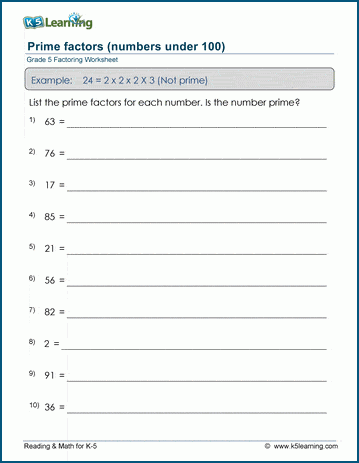# Prime factors (< 100)

## Math worksheets: Prime factors of numbers less than 100

Below are six versions of our grade 5 math worksheet on determining prime factors. A prime number can only be divided evenly  only by 1 and itself.  In these exercises students are asked to find the set of prime numbers which multiply together to give the original number. Numbers up to 100 are considered. These worksheets are pdf files.## More factoring & prime factors worksheets

Explore all of our factoring worksheets, from factoring numbers under 50 to prime factor trees, GCFs and LCMs.

What is K5?

K5 Learning offers free worksheets, flashcards and inexpensive workbooks for kids in kindergarten to grade 5.  We help your children build good study habits and excel in school.